# Chapter 3 Simple Random Sampling

In a simple random sample without replacement (SRSWOR) of size $$n$$ from a population of size $$N$$, every possible combination of $$n$$ distinct population members has an equal chance of selection. This also means that the probability that any individual population member is selected is $$\pi=n/N$$, and the weight each sample member carries in inference is $$w=N/n$$.

## 3.1 Drawing a SRSWOR

If we have a dataset loaded we can use iNZight to select a SRSWOR from it:

• Go Dataset > Filter > randomly then Proceed
• Specify the Sample Size $$n$$ (must be less than or equal to the number of rows in the dataset), and only ask for 1 sample.
• iNZight creates a new dataset with .filtered added to the end of the dataset name

You can choose a single sample of $$n$$ rows, or multiple samples of $$n$$ rows. If you choose more than one sample then iNZight creates a single dataset, but adds a final column Sample.Number which indicates which sample each row belongs to. If you ask for $$m=10$$ samples of $$n=50$$ rows then you will have a filtered dataset that is 500 lines long. Each set of 50 rows is a new sample, and note that although all of the members within an individual sample are distinct, it is possible for an individual to appear in more than one sample.

## 3.2 Properties of a SRSWOR

The number of possible samples of size $$n$$ that can be selected from a population of size $$N$$ can be computed using R code in the R Console using the function choose(N,n) which evaluates the Binomial Coefficient: $\binom{N}{n} = {}^NC_n = \frac{N!}{n!(N-n)!}$

Example: If $$N=6$$ and $$n=3$$ then the number of possible simple random samples can be computed by typing in the R Console window:

> choose(6,3)
 20

In SRSWOR the probability of selection $$\pi_i$$ of a population member is $\pi_i = \frac{n}{N}$ The sample weights are given by $w_i = \frac{1}{\pi_i} = \frac{N}{n}$

Example: If $$N=6$$ and $$n=3$$ then the probability of selection is

> 3/6
 0.5

and the sample weights are

> 6/3
 2

That is to say, each person in the sample represents 2 people from the population: themselves and 1 other.

## 3.3 Sample Size Calculation

• Estimating a mean: If the desired Margin of error for an estimate of the populaton mean is $$m$$, and an estimate of the standard deviation of the variable is $$s_y$$ then first compute $n' = \left(\frac{Z^\ast}{m}\right)^2 s_y^2$ where $$Z^\ast=1.96$$ for 95% Confidence. The compute the actual sample size required by applying the finite population correction: $n = \frac{n'}{1+\frac{n'}{N}}$ (the + sign in the denominator is correct here).

• Estimating a proportion: If the desired Margin of error for an estimate of the population proportion is $$m$$, and a rough prior estimate of the population proportion is $$p$$ then first compute $n' = \left(\frac{Z^\ast}{m}\right)^2 p(1-p)$ where $$Z^\ast=1.96$$ for 95% Confidence. The compute the actual sample size required by applying the finite population correction: $n = \frac{n'}{1+\frac{n'}{N}}$ If there is no good prior estimate of $$p$$, set $$p=0.5$$ in the above.

Example: In a population of size $$N=10000$$ estimate the proportion of people who have a disability to a Margin of Error of $$\pm 2\%$$. A prior estimate of the proportion of people in the population with a disability is 0.25. $\begin{eqnarray*} n' &=& \left(\frac{1.96}{0.02}\right)^2 (0.25)(1-0.25) = 1800.75\\ n &=& \frac{1800.75}{1 + \frac{1800.75}{10000}} = 1526 \end{eqnarray*}$ At the R console:

> ndash <- (1.96/0.02)^2 * 0.25*(1-0.25)
> n <- ndash/(1+ndash/10000)
> n
 1525.962

## 3.4 Specifying a SRSWOR design

In order for iNZight to carry out an appropriate analysis of a SRSWOR from a finite population, we need to specify the population size $$N$$. In iNZight this means

• adding a column to the dataset with the population size repeated in every entry of the column, and then
• telling iNZight that the data should be treated as a SRSWOR, and specifying the column with the population size.

• Variables > Create new variables
• Fill in the two boxes in the window that opens:
• in the left hand box replace new.variable with the name you’re giving the population size: e.g. N
• in the right hand box fill in the value: e.g. 6194 for the apisrs data set
• Click SUBMIT

To tell iNZight to use this column:

• Dataset > Survey Design > Specify Design
• In the Finite population correction box choose the name of the population size variable
• Click OK

Note that we can clear the specification of a sample design just by going

• Dataset > Survey Design > Remove Design

## 3.5 Simple Estimates

After choosing a variable or variable to display, the plot that shown alters to take account of the specified design, and Get Summary and Get Inference also have modified outputs.

### 3.5.1 Example - Single numerical variable

If we load the apisrs dataset note that it actually already has a population size column included, named fpc. If we specify this as the population size variable and then choose the enroll variable the default plot changes from a box+dot plot to a boxplot+histogram.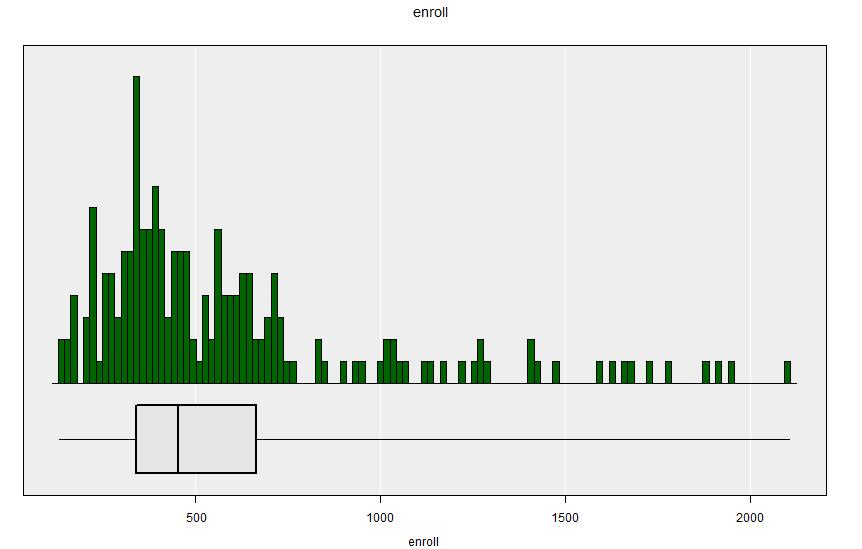Distribution of “enroll” in the apisrs dataset - including the finite population correction.

Get Summary reports details of the population size, and instead of just reporting single values for the variable’s characteristics (mean, standard deviation, median, quartiles) it repors these values as estimates and also reports their standard errors.

====================================================================================================
iNZight Summary - Survey Design
----------------------------------------------------------------------------------------------------
Primary variable of interest: enroll (numeric)

Total number of observations: 200
Estimated population size: 6194
----------------------------------------------------------------------------------------------------
Independent Sampling design
survey::svydesign(id = ~ 1, fpc = ~ N, data = dataSet)
====================================================================================================

Summary of enroll:
------------------

Population estimates:

25%   Median      75%     Mean       SD     Total   Est. Pop. Size   |   Sample Size   Min    Max
339.000   453.00   664.00   584.61   393.45   3621074             6194   |           200   131   2106

Standard error of estimates:

9.632    28.53    28.51    27.37    30.39    169520                0

Design effects:

1.00                  1

====================================================================================================

This summary output gives the estimate not only of the population mean school size $$\widehat{\bar{Y}}=584.61$$ but also the population total: $$\widehat{Y}=N\widehat{\bar{Y}}=3621074$$: an estimate of the total population of all the schools in the population combined. The standard errors of these two estimates are given ($$30.39$$ and $$169520$$ respectively).

The RSE for the estimate of the mean is the ratio of the standard error to the estimate: $\begin{eqnarray*} \mathbf{RSE}[\widehat{\bar{Y}}] &=& \frac{\mathbf{SE}[\widehat{\bar{Y}}]}{\widehat{\bar{Y}}} \end{eqnarray*}$ In the R Console:

> 27.27/584.61
 0.0466465

The Design Effect (Deff) is the variance of the estimate divided by the variance that would have been achieved by using data from a SRSRWOR of the same sample size. So by definition the Deff for a SRSWOR is always 1.

The output of Get Inference looks very similar to before, except that the population size is given.

====================================================================================================
iNZight Inference using Normal Theory
----------------------------------------------------------------------------------------------------
Primary variable of interest: enroll (numeric)

Total number of observations: 200
Estimated population size: 6194
----------------------------------------------------------------------------------------------------
Independent Sampling design
survey::svydesign(id = ~ 1, fpc = ~ N, data = dataSet)
====================================================================================================

Inference of enroll:
--------------------

Population Mean with 95% Confidence Interval

Lower    Mean   Upper
531   584.6   638.3

====================================================================================================

Note however that the although the estimated mean is the same as before (584.6) the confidence interval is just slightly narrower: it is now (531.0,638.3) whereas without specifying the population size it was (529.7, 584.6). This is due to the variance of an estimator of the mean with SRSWOR including the finite population correction (fpc): $\begin{eqnarray*} \text{Var}[\widehat{\bar{Y}}] &=& \left(1-\frac{n}{N}\right)\frac{S_Y^2}{n} \end{eqnarray*}$ and the fpc factor $$(1-n/N)$$ reduces the variance, thereby reducing the margin of error.

A confidence interval for the population total can be computed using the R Console by multiplying the confidence interval for the mean by the population size $$N=6194$$:

> 6194*c(531, 638.3)
 3.289014\times 10^{6}, 3.9536302\times 10^{6}

### 3.5.2 Example - Single categorical variable

Now look at the School Type variable stype: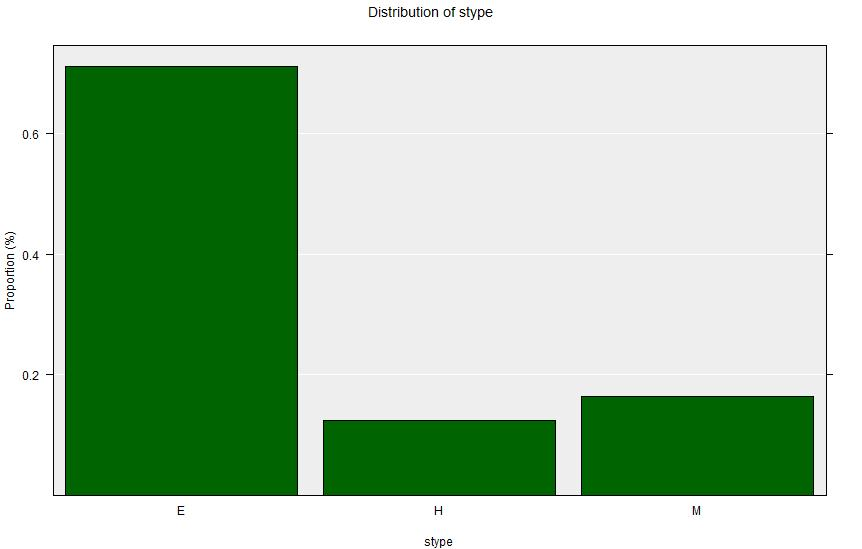Distribution of “stype” in the apisrs dataset - including the finite population correction.

Summary output:

====================================================================================================
iNZight Summary - Survey Design
----------------------------------------------------------------------------------------------------
Primary variable of interest: stype (categorical)

Total number of observations: 200
Estimated population size: 6194
----------------------------------------------------------------------------------------------------
Independent Sampling design
survey::svydesign(id = ~ 1, fpc = ~ fpc, data = dataSet)
====================================================================================================

Summary of the distribution of stype:
-------------------------------------

Population Estimates:

E        H        M   Total
Count      4398      774     1022    6194

Percent    71.00%   12.50%   16.50%    100%
std err     3.16%    2.31%    2.59%

Design effects      1.00     1.00     1.00

====================================================================================================

Inference Output

====================================================================================================
iNZight Inference using Normal Theory
----------------------------------------------------------------------------------------------------
Primary variable of interest: stype (categorical)

Total number of observations: 200
Estimated population size: 6194
----------------------------------------------------------------------------------------------------
Independent Sampling design
survey::svydesign(id = ~ 1, fpc = ~ fpc, data = dataSet)
====================================================================================================

Inference of the distribution of stype:
---------------------------------------

Estimated Population Proportions with 95% Confidence Interval

Lower   Estimate   Upper
E   0.6480      0.710   0.772
H   0.0798      0.125   0.170
M   0.1143      0.165   0.216

### Differences in proportions of stype
(col group - row group)

Estimates

E       H
H   0.585
M   0.545   -0.04

95% Confidence Intervals

E          H
H   0.5636
0.6063
M   0.5219   -0.05634
0.5681   -0.02366

====================================================================================================

## 3.6 Two-variable analyses

The procedure for two variables with a SRSWOR design are the same as they are without a sample design. The output is slightly different - in particular for estimated counts of categorical variables: they are estimates of population total counts, and not simply sample counts.

### 3.6.1 Two numerical variables

Here we investigate the relationship between overall school academic performance (api00) and proportion of children receiving school meals (meals) in the apisrs data set.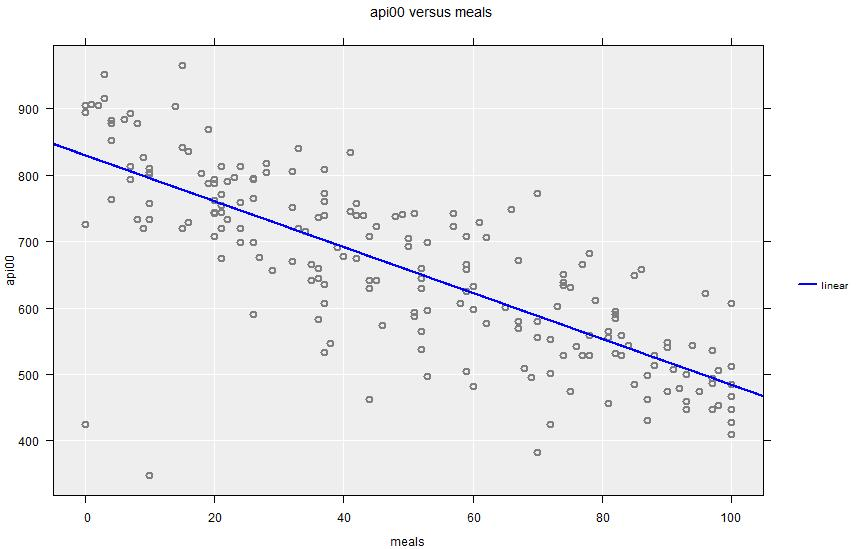The Summary Output looks similar to the standard output, except that in addition to the correlation coefficient it also gives the coefficients for a linear regression fit. The Inference output also gives confidence intervals for those coefficients.

Summary Output:

====================================================================================================
iNZight Summary - Survey Design
----------------------------------------------------------------------------------------------------
Response/outcome variable: api00 (numeric)
Predictor/explanatory variable: meals (numeric)

Total number of observations: 200
Estimated population size: 6194
----------------------------------------------------------------------------------------------------
Independent Sampling design
survey::svydesign(id = ~ 1, fpc = ~ fpc, data = dataSet)
====================================================================================================

Summary of api00 versus meals:
------------------------------

Summary of api00 versus meals:
------------------------------

Correlation: -0.78  (using Pearson's Correlation)

====================================================================================================

Inference Output:

====================================================================================================
iNZight Inference using Normal Theory
----------------------------------------------------------------------------------------------------
Response/outcome variable: api00 (numeric)
Predictor/explanatory variable: meals (numeric)

Total number of observations: 200
Estimated population size: 6194
----------------------------------------------------------------------------------------------------
Independent Sampling design
survey::svydesign(id = ~ 1, fpc = ~ fpc, data = dataSet)
====================================================================================================

Inference of api00 versus meals:
--------------------------------

Linear Trend Coefficients with 95% Confidence Intervals

Estimate     Lower     Upper   p-value
Intercept     829.37    802.22    856.52    <2e-16
meals     -3.455   -3.8662   -3.0437    <2e-16

p-values for the null hypothesis of no association, H0: beta = 0

====================================================================================================

### 3.6.2 One numerical and one categorical variable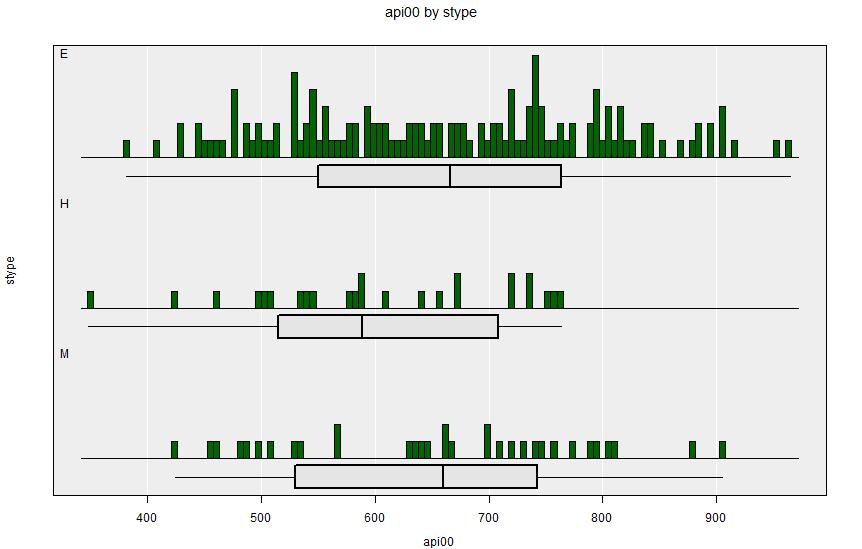Summary Output:

====================================================================================================
iNZight Summary - Survey Design
----------------------------------------------------------------------------------------------------
Primary variable of interest: api00 (numeric)
Secondary variable: stype (categorical)

Total number of observations: 200
Estimated population size: 6194
----------------------------------------------------------------------------------------------------
Independent Sampling design
survey::svydesign(id = ~ 1, fpc = ~ fpc, data = dataSet)
====================================================================================================

Summary of api00 by stype:
--------------------------

Population estimates:

25%   Median      75%       Mean       SD         Total   Est. Pop. Size   |   Sample Size   Min   Max
E   550.50   666.00   763.50   666.1408   135.73   2929514.240           4397.7   |           142   382   965
H   515.00   589.00   708.50   605.3600   113.46    468699.980            774.2   |            25   348   764
M   530.25   660.00   742.75   654.2727   129.11    668673.270           1022.0   |            33   425   905

Standard error of estimates:

E    13.91    19.63    15.54    11.1935     5.90    139532.264            196.0
H    39.56    33.05    34.43    21.9266    12.90     88125.597            142.8
M    41.11    37.92    25.31    21.8261    11.41    107242.153            160.3

Design effects:

E                                0.9979                  8.018
H                                0.9648                 25.998
M                                0.9746                 22.526

====================================================================================================

Inference Output:

====================================================================================================
iNZight Inference using Normal Theory
----------------------------------------------------------------------------------------------------
Primary variable of interest: api00 (numeric)
Secondary variable: stype (categorical)

Total number of observations: 200
Estimated population size: 6194
----------------------------------------------------------------------------------------------------
Independent Sampling design
survey::svydesign(id = ~ 1, fpc = ~ fpc, data = dataSet)
====================================================================================================

Inference of api00 by stype:
----------------------------

Population Means with 95% Confidence Intervals

Lower    Mean   Upper
E   644.2   666.1   688.1
H   562.4   605.4   648.3
M   611.5   654.3   697.1

Wald test for stype (ANOVA equivalent for survey design)

F = 3.0482, df = 2 and 197, p-value = 0.04969

Null Hypothesis: true group means are all equal
Alternative Hypothesis: true group means are not all equal

### Difference in mean api00 between stype groups
(col group - row group)

Estimates

E        H
H   60.78
M   11.87   -48.91

====================================================================================================

### 3.6.3 Two categorical variables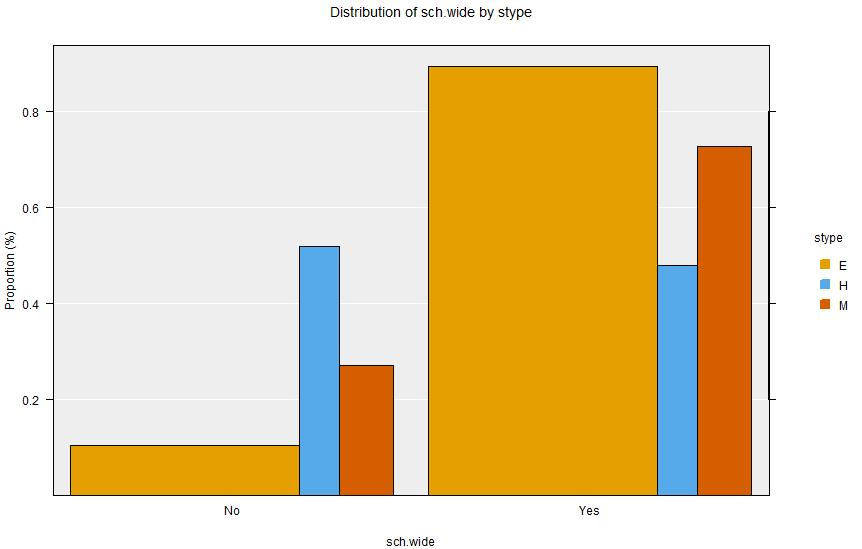Summary Output:

====================================================================================================
iNZight Summary - Survey Design
----------------------------------------------------------------------------------------------------
Primary variable of interest: sch.wide (categorical)
Secondary variable: stype (categorical)

Total number of observations: 200
Estimated population size: 6194
----------------------------------------------------------------------------------------------------
Independent Sampling design
survey::svydesign(id = ~ 1, fpc = ~ fpc, data = dataSet)
====================================================================================================

Summary of the distribution of sch.wide (columns) by stype (rows):
------------------------------------------------------------------

Table of Estimated Population Counts:

No    Yes   Row Total
E   465   3933        4398
H   403    372         775
M   279    743        1022

Table of Estimated Population Percentages:

No     Yes   Total   Row N
E   10.6%   89.4%    100%    4398
H     52%     48%    100%     775
M   27.3%   72.7%    100%    1022

Standard error of estimated percentages:

No     Yes
E   2.544   2.544
H   9.854   9.854
M   7.646   7.646

Design effects:

No     Yes
E   0.998   0.998
H   0.965   0.965
M   0.975   0.975

====================================================================================================

Inference Output:

====================================================================================================
iNZight Inference using Normal Theory
----------------------------------------------------------------------------------------------------
Primary variable of interest: sch.wide (categorical)
Secondary variable: stype (categorical)

Total number of observations: 200
Estimated population size: 6194
----------------------------------------------------------------------------------------------------
Independent Sampling design
survey::svydesign(id = ~ 1, fpc = ~ fpc, data = dataSet)
====================================================================================================

Inference of the distribution of sch.wide (columns) by stype (rows):
--------------------------------------------------------------------

Estimated Proportions

No     Yes   Row sums
E   0.106   0.894          1
H   0.520   0.480          1
M   0.273   0.727          1

95% Confidence Intervals

No     Yes
E   0.0558   0.845
0.1555   0.944
H   0.3269   0.287
0.7131   0.673
M   0.1229   0.577
0.4226   0.877

Chi-square test for equal distributions

X^2 = 13.482, df = 2 and 398, p-value = 2.1606e-06

Null Hypothesis: distribution of sch.wide does not depend on stype
Alternative Hypothesis: distribution of sch.wide changes with stype

### Differences in proportions of stype with the specified sch.wide

# Group differences between proportions with: sch.wide = No
(col group - row group)

Estimates

E         H
H   0.4144
M   0.1671   -0.2473

# Group differences between proportions with: sch.wide = Yes
(col group - row group)

Estimates

E        H
H   -0.4144
M   -0.1671   0.2473

====================================================================================================